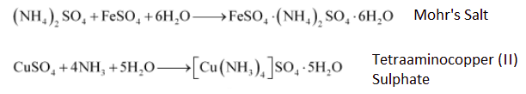Q

# FeSO_{4} solution mixed with (NH_{4})_{2}SO_{4} solution in 1:1 molar ratio gives the test of Fe^{2+} ion but CuSO_{4} solution mixed with aqueous ammonia in 1:4 molar ratio does not give the test of Cu^{2+} ion. Explain why?

9.2  solution mixed with solution in 1:1 molar ratio gives the test of ion but   solution mixed with aqueous ammonia in 1:4 molar ratio does not give the test of  ion. Explain why?